## How to Solve the Thales Theorem?

• To prove the Thales theorem, draw a perpendicular bisector of ∠
• Let point M be midpoint point of line AC
• Also let ∠MBA = ∠BAM = β and ∠MBC =∠BCM
• Line AM = MB = MC = the radius of the circle.
• ΔAMB and ΔMCB are isosceles triangles.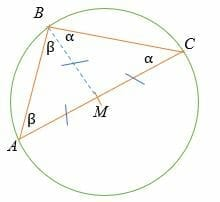By triangle sum theorem,

BAC +∠ACB +∠CBA = 180°

β + β + α + α = 180°

Factor the equation.

2 β + 2 α = 180°

2 (β + α) = 180°

Divide both sides by 2.

β + α = 90°.

Therefore, ∠ABC = 90°, hence proved

Let’s work out a few example problems involving Thales theorem.

Example 1

Given that point O is the center of the circle shown below, find the value of x.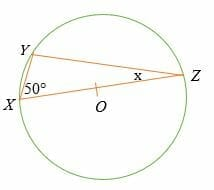Solution

Given that line, XY is the diameter of the circle, then by Thales theorem,

XYZ = 90°.

Sum of interior angles of a triangle = 180°

90° + 50° + x =180°

Simplify.

140° + x =180°

Subtract 140° on both sides.

x = 180° – 140°

x = 40°.

So, the value of x is 40 degrees.

Example 2

If point D is the centre of the circle shown below, calculate the diameter of the circle.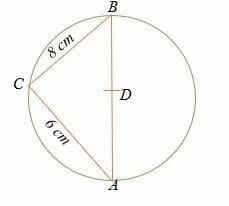Solution

By Thales theorem, triangle ABC is a right triangle where ∠ACB = 90°.

To find the diameter of the circle, apply the Pythagorean theorem.

CB2 + AC2 =AB2

82 + 62 = AB2

64 + 36 = AB2

100 = AB2

AB = 10

Hence, the diameter of the circle is 10 cm

Example 3

Find the measure of angle PQR in the circle shown below. Assume point R is the centre of the circle.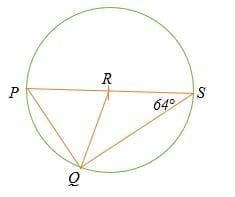Solution

Triangle RQS and PQR are isosceles triangles.

RQS =∠RSQ =64°

By Thales theorem, ∠PQS = 90°

So, ∠PQR 90° – 64°

= 26°

Hence, the measure of angle PQR is 26°.

Example 4

Which one of the following statements is true about definition of the Thales theorem?

A. The central angle is twice the measure of the inscribed angle

B. An angle inscribed in a half-circle will be a right angle.

C. The diameter of a circle is the longest chord.

D. The diameter of a circle is twice the length of the radius.

Solution

B. An angle inscribed in a half-circle will be a right angle.

Example 5

In the circle shown below, line AB is the diameter of the circle with centre C.

1. Find the measure of ∠ BCE
2. ∠ DCA
3. ∠ ACE
4. ∠ DCB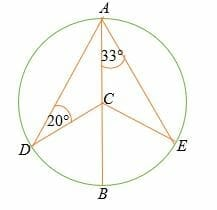Solution

Given triangle ACE is an isosceles triangle,

∠ CEA =∠ CAE = 33°

So, ∠ ACE =180° – (33° + 33°)

∠ ACE = 114°

But angles on a straight = 180°

Hence, ∠ BCE = 180° – 114°

= 66°

Triangle ADC is an isosceles triangle, therefore, ∠ DAC =20°

By triangle sum theorem, ∠DCA = 180° – (20° + 20°)

∠ DCA = 140°

∠ DCB = 180° – 140°

= 40°

Example 6

What is the measure of ∠ABC?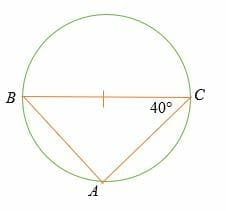Solution

The Thales theorem states that BAC = 90°

And by triangle sum theorem,

ABC + 40° + 90° = 180°

ABC = 180° – 130°

= 50°

Example 7

Find the length of AB in the circle shown below.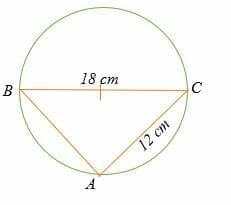Solution

Triangle ABC is a right triangle.

Apply the Pythagorean theorem to find length AB.

AB2 + 122 = 182

AB2 + 144 = 324

AB2 = 324 – 144

AB2 = 180

AB = 13.4

Therefore, the length of AB is 13.4 cm.